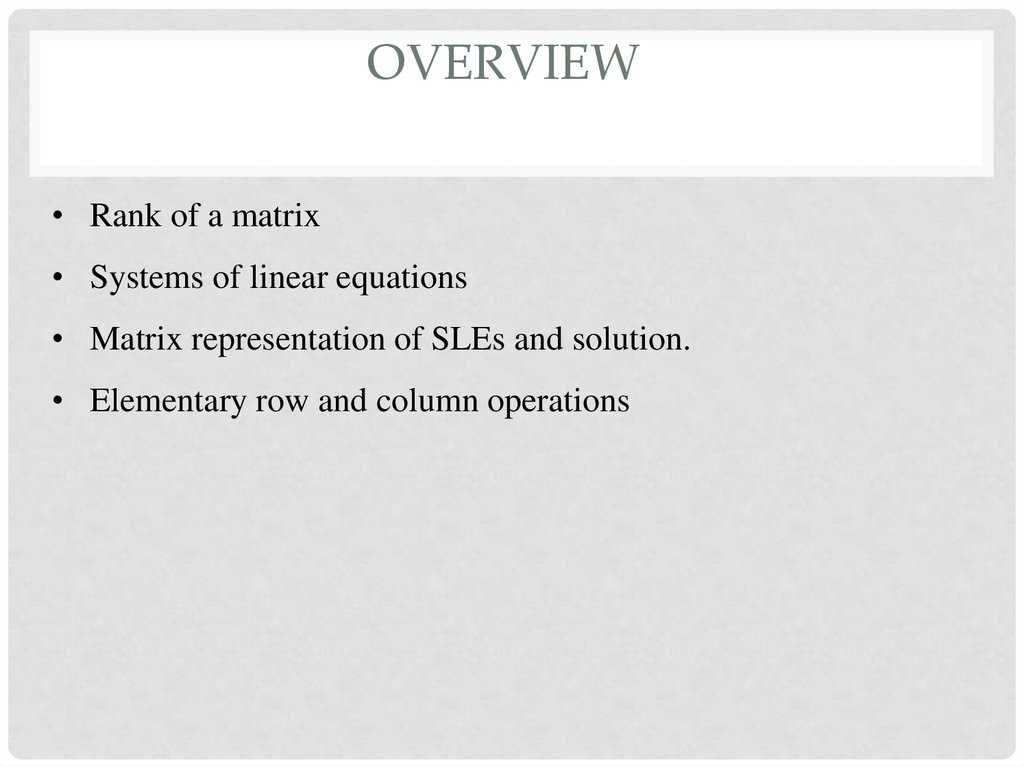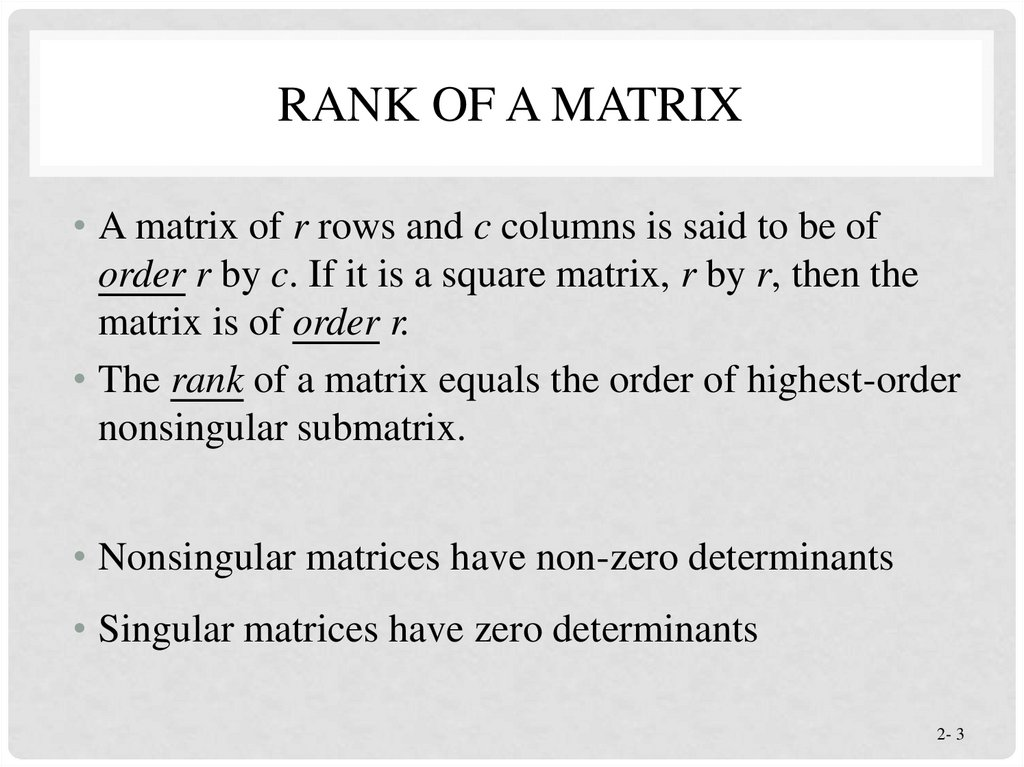System of linear equations. Lecture 4-5

1. System of linear equations

SYSTEM OF LINEAR
EQUATIONS
K A R A S H B AY E VA Z H . O .
SENIOR-LECTURER

2. overview

OVERVIEW
• Rank of a matrix
• Systems of linear equations
• Matrix representation of SLEs and solution.
• Elementary row and column operations

3. Rank of A Matrix

RANK OF A MATRIX
• A matrix of r rows and c columns is said to be of
order r by c. If it is a square matrix, r by r, then the
matrix is of order r.
• The rank of a matrix equals the order of highest-order
nonsingular submatrix.
• Nonsingular matrices have non-zero determinants
• Singular matrices have zero determinants
2- 3

5. Computing rank by various methods

COMPUTING RANK BY VARIOUS
METHODS
• By Gauss elimination
• By determinants
• By minors

7. Elementary row and column operations

ELEMENTARY ROW AND COLUMN OPERATIONS

8. Elementary row and column operations

ELEMENTARY ROW AND COLUMN OPERATIONS

12. Example 1: Rank of Matrix

EXAMPLE 1: RANK OF MATRIX
2 3 order matrix,
1 2 4
R
2
4
8
3 square submatrices:
1 2
1 4
R1
, R2
,
2 4
2 8
2 4
R3
4
8
Each of these has a determinant of 0, so the rank is less than 2.
Thus the rank of R is 1.
2- 12

13. Example 2: Rank of Matrix

EXAMPLE 2: RANK OF MATRIX
2
A 1
9
4
3
10
6
5
11
Since |A|=0, the rank is not 3. The following
submatrix has a nonzero determinant:
2 4
2(3) 4(1) 2
1 3
Thus, the rank of A is 2.
2- 13

14. Systems of linear equations

SYSTEMS OF LINEAR EQUATIONS

15.

MATRIX REPRESENTATION OF SLES
• Any SLEs can be formulated in the matrix form:

16. Methods of solving SLE

METHODS OF SOLVING SLE

17. Methods of solving SLE

METHODS OF SOLVING SLE

18. Gauss elimination

GAUSS ELIMINATION
• Two steps
• 1. Forward Elimination
• 2. Back Substitution

19. Forward Elimination

FORWARD ELIMINATION
A set of n equations and n unknowns
a11x1 a12 x2 a13 x3 ... a1n xn b1
a21x1 a22 x2 a23 x3 ... a2n xn b2
.
.
.
.
.
.
an1x1 an 2 x2 an3 x3 ... ann xn bn
(n-1) steps of forward elimination

20. Forward Elimination

FORWARD ELIMINATION
Step 1
For Equation 2, divide Equation 1 by a11 and
multiply by a21 .
a21
a (a11x1 a12 x2 a13 x3 ... a1n xn b1 )
11
a21
a21
a21
a21x1
a12 x2 ...
a1n xn
b1
a11
a11
a11

21. Forward Elimination

FORWARD ELIMINATION
Subtract the result from Equation 2.
a21x1 a22 x2 a23 x3 ... a2n xn b2
a21
a21
a21
− a21x1 a a12 x2 ... a a1n xn a b1
11
11
11
_________________________________________________
a21
a21
a21
a22
a12 x2 ... a2 n
a1n xn b2
b1
a11
a11
a11
or
a x ... a x b
'
22 2
'
2n n
'
2

22. Forward Elimination

FORWARD ELIMINATION
Repeat this procedure for the remaining
equations to reduce the set of equations as
a11x1 a12 x2 a13 x3 ... a1n xn b1
'
'
a22
x2 a23
x3 ... a2' n xn b2'
'
'
a32
x2 a33
x3 ... a3' n xn b3'
.
.
.
.
.
.
.
.
.
'
an' 2 x2 an' 3 x3 ... ann
xn bn'
End of Step 1

23. Forward Elimination

FORWARD ELIMINATION
Step 2
Repeat the same procedure for the 3rd term of
Equation 3.
a11x1 a12 x2 a13 x3 ... a1n xn b1
'
'
a22
x2 a23
x3 ... a2' n xn b2'
"
a33
x3 ... a3" n xn b3"
.
.
.
.
.
.
"
an" 3 x3 ... ann
xn bn"
End of Step 2

24. Forward Elimination

FORWARD ELIMINATION
At the end of (n-1) Forward Elimination steps, the
system of equations will look like
a11 x1 a12 x2 a13 x3 ... a1n xn b1
'
'
a22
x2 a23
x3 ... a2' n xn b2'
"
a33
x3 ... a3" n xn b3"
.
.
.
.
.
.
n 1
n 1
ann
xn bn
End of Step (n-1)

25. Matrix Form at End of Forward Elimination

MATRIX FORM AT END OF
FORWARD ELIMINATION
a11 a12
0 a'
22
0
0
0
0
a13
'
a23
a"33
0
a1n x1 b1
'
'
a 2 n x2
b2
a"3n x3 b3"
(n 1 )
xn bn(n-1 )
0 ann

26. Back Substitution Starting Eqns

BACK SUBSTITUTION STARTING EQNS
a11 x1 a12 x2 a13 x3 ... a1n xn b1
'
'
a22
x2 a23
x3 ... a2' n xn b2'
"
a33
x3 ... an" xn b3"
.
.
.
n 1
.
.
.
n 1
ann xn bn

27. Back Substitution

BACK SUBSTITUTION
( n 1)
n
( n 1)
nn
b
xn
a

28. Back Substitution

BACK SUBSTITUTION
( n 1)
n
( n 1)
nn
b
xn
a
xi
bi i 1 ai ,ii 11 xi 1 ai ,ii 12 xi 2 ... ai ,in 1 xn
i 1
aii
i 1
xi
bi
aij i 1 x j
n
j i 1
i 1
ii
a
for i n 1,...,1
for i n 1,...,1

32. Existence and Uniqueness of Solutions

EXISTENCE AND UNIQUENESS OF SOLUTIONS

33. Existence and Uniqueness of Solutions

EXISTENCE AND UNIQUENESS OF SOLUTIONS

THE END